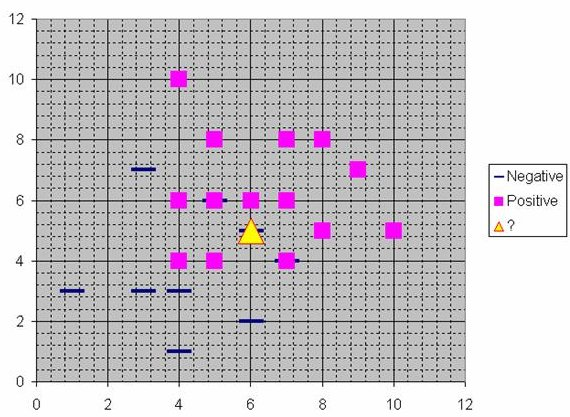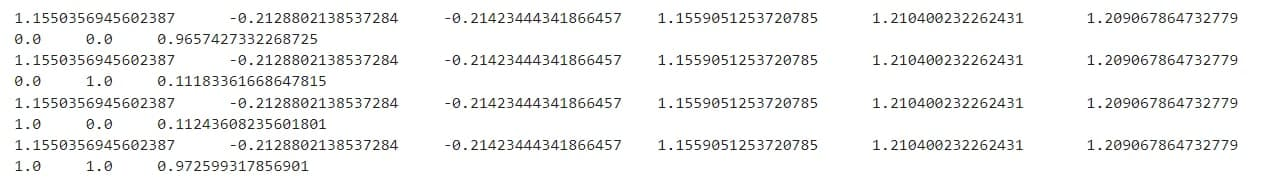##### What is Radial Basis Function Neural Network?

The radial basis function network is one of the most widely used types of artificial neural networks for Approximation of functions. A radial basis function network has higher learning speed and universal approximation than other types of neural networks.

An RBF network consists of three layers of feedforward neurons. This network consists of three layers: the first represents the network inputs, the second is the hidden layer containing non-linear RBF activation units, and the third represents the network output. Gaussian functions are most commonly used in RBFNs as activation functions.

The RBF network construction technique is similar to K-Means clustering and PNN/GRNN networks. RBF networks have a variable number of neurons, usually much less than the number of training points, whereas PNN/GRNN networks have one neuron for each point in the training file. A PNN/GRNN network is usually more accurate than a RBF network when dealing with small or medium-sized training sets, but PNN/GRNN networks are impractical when dealing with large training sets.##### Working of Radial Basis Function networks

RBF neural networks are conceptually similar to K-Nearest Neighbours , even though they are implemented differently. Generally, when a predicted target value is close to the actual value of the predictor variables, it is highly likely that the predicted target value will be similar to that of other items. See figure below.Consider two predictor variables, x and y, for each case in the training set. Figure 1 shows the coordinates of the cases plotted using x and y values. Assume that the target variable consists of two categories; positive and negative, denoted by squares and dashes, respectively. Consider the following situation: Suppose we are predicting the value of a new case represented by the triangle with predictor values x=6, y=5.1. Does it make sense to predict the target as positive or negative?

Almost exactly on top of the triangle is a dash symbolizing a negative value. Yet that dash occupies an odd position in comparison to the other dashes, which cluster below the squares and to the left. If that were the case, then the negative value might be considered an anomaly.

Based on how many neighboring points are considered in this example, the nearest neighbor classification will be determined. The new point would appear negative if 1-NN was used and only the closest point was taken into consideration since it is on top of a known negative point. As an alternative, if 9-NN classification is used and the closest 9 points are taken into account, then the 8 points surrounding the close negative point could counterbalance its effect.

Using an RBF network, the RBF neurons are placed according to the space described by the predictor variables (x,y in the following example). The dimensions of the space are the same number as the predictor variables. To compute each neuron’s weight (influence), a radial basis function (RBF) (also called a kernel function) is applied to the distance between the point being evaluated (in this case, the triangle). The radial basis function gets its name from its argument, which is the radius distance.

Weight = RBF(distance)

Neurons have less influence the further they are from the point being evaluatedRadial basis functions can take many forms, but Gaussian functions are most commonly used:It has as many dimensions as there are variables in the RBF function if there are more than one predictor variable. An example of three neurons plotted on a space containing two predictor variables, X and Y, is presented below. Using the RBF functions to determine the value of Z:Using RBF functions multiplied by weights calculated for each neuron, the best predicted value of the new point is obtained.Typically, radial basis functions for neurons are defined by a center and a radius (also called a spread). Different neurons will have varying radiuses.

With a larger spread, neurons are more likely to influence a point at a distance.###### RBF Network Architecture

Networks with RBF are composed of three layers:

Input Layer : Each predictor variable is represented by a neuron in the input layer. Taking categorical variables into account, N-1 neurons are used where N is the number of categories. By subtracting the median value from the range of values, and dividing by the interquartile range, the input neurons normalize the range of values. Next, each of the hidden layer neurons receives the values from the input neurons.

Hidden layer – The number of neurons in this layer varies (the optimal number is determined by the training process). Neurons are made up of radial basis functions centered around a point with as many dimensions as there are predictor variables. RBF functions may have different spreads (radii) based on their dimensionality. Training determines their centers and spreads. The hidden neuron uses the spread values of the x vector of input values from the input layer to compute the Euclidean distance of the test case. This distance is then applied to this distance using the RBF kernel function. Once the kernel function has been applied, a final summation value is obtained.

Summation layer – A neural output in the hidden layer is multiplied by the weight associated with it (in this example, W1, W2, …, Wn) and passed on to the network’s summation function. This function adds up the weighted values and displays the resulting value as the network’s output. The bias value of 1.0 is not shown in this figure since it is multiplied by a weight W0 and fed into the summation layer.  Each target category in a classification problem has a single output. The value output for a category is its probability that the case under consideration falls into that category##### Training the RBNN

⁃ To begin, back propagation should be used to train the hidden layer.

⁃ Neural Network training(back propagation) is a curve fitting method. During the training phase, a non-linear curve is fitted. Stochastic approximation is used, which is called back propagation.

⁃ For each of the node in the hidden layer, we have to find t(receptors) & the variance (σ)[variance — the spread of the radial basis function]

⁃ The weighting vectors between hidden and output layers need to be updated on the second training phase.

⁃ Each node within the hidden layer corresponds to a transformation basis function. It is possible to have non-linear separability with any of the functions OR with any combination of the functions.

Therefore, we include all non-linearity terms in our hidden layer transformation. A hypersurface equation would be comprised of X2 + Y2 + 5XY (where X & Y are inputs).

A clustering algorithm is used in the first stage of training. Cluster centers are defined as needed. We design cluster centers based on the clustering algorithm, which we assign as receptors to each hidden neuron.

Using N samples or observations, I will cluster them into M clusters (N > M).

Consequently, the output “clusters” are the “receptors”.

⁃ The variance of each receptor can be calculated as “the squared sum of the distance between the receptor and the nearest sample in each cluster” := 1/N * ||X — t||²

⁃ The first training phase interprets the feature vector as a feature projection onto the transformed space.##### Advantages of using RBNN over the MLP

There are several advantages to using RBNN over MLP:

1. Training in RBNN is faster than in Multilayer Perceptron (MLP), which requires many interactions.
2. Each node in the hidden layer of the RBNN can be easily interpreted for its meaning or function. MLP makes it difficult to do so.
3. MLP makes it difficult to parameterize (number of nodes in hidden layer & number of hidden layers). The RBNN does not have this problem.
4. As a result, RBNN requires more time for classification than MLP.

#### Coding

Here we are going to train the model by pseudo inverse technique to demonstrate Radial Based Function Neural Network.

				
// here we are creating a class which contains handles arrays of input and output layer
class Net {
var inputs =
arrayOf(doubleArrayOf(0.0, 0.0), doubleArrayOf(0.0, 1.0), doubleArrayOf(1.0, 0.0), doubleArrayOf(1.0, 1.0))
var outputs = doubleArrayOf(1.0, 0.0, 0.0, 1.0)
var net = arrayOfNulls(2)
init {  // initializing the network of arrays
net = Unit(0.5, doubleArrayOf(0.0, 0.0), 0.5)
net = Unit(0.5, doubleArrayOf(1.0, 1.0), 0.5)
}



Here in this block we have created the function to train our model and trying to predict the outcome.

				

fun train() {
for (i in inputs.indices) {
val output = outputs[i]
var predictedoutput = 0.0
for (j in inputs[i].indices) {
predictedoutput += net[j]!!.phi(inputs[i]) * net[j]!!.w
}
//predictedoutput= Math.round(predictedoutput);
for (j in inputs[i].indices) {
net[j]!!.update(inputs[i], output, predictedoutput)
}
}
}



Declared a function which deals with araay further for testing purpose.

				

fun test(inputs: DoubleArray) {
var predictedOutput = 0.0
for (i in inputs.indices) {
predictedOutput += net[i]!!.phi(inputs) * net[i]!!.w
print(net[i]!!.w.toString() + "\t" + net[i]!!.c + "\t" + net[i]!!.c + "\t")
}
println()
// predicting the output by roundup
for (i in inputs.indices) {
print(inputs[i].toString() + "\t")
}
print(predictedOutput)
println()
}



this class operates unit which calculate distance for the model.

				

class Unit(var sigma: Double, var c: DoubleArray, var w: Double) {
var n1 = 0.1
var n2 = 0.1
fun phi(input: DoubleArray): Double {
var distance = 0.0
for (i in c.indices) distance += Math.pow(input[i] - c[i], 2.0)
return Math.pow(Math.E, -distance / (2 * Math.pow(sigma, 2.0)))
}



Function append the updated values in the array and generate different output

				

fun update(input: DoubleArray, desired: Double, output: Double) {
val phi = phi(input)
val diffOutput = desired - output
for (i in c.indices) c[i] = c[i] + n1 * diffOutput * w * phi * (input[i] - c[i]) / (sigma * sigma)
w = w + n2 * diffOutput * phi
}
}}



Here we are passing the trained model and trying to predict the outcome

				
var abc=Net() // declaring variable for network model
for (i in 0..99) {
abc.train()
}


				
import kotlin.jvm.JvmStatic
abc.test(doubleArrayOf(0.0, 0.0))
abc.test(doubleArrayOf(0.0, 1.0))
abc.test(doubleArrayOf(1.0, 0.0))
abc.test(doubleArrayOf(1.0, 1.0)) // testing the model



#### OutputWith this we are done with this set of example, hope you have learned something new. Thank you for your time.